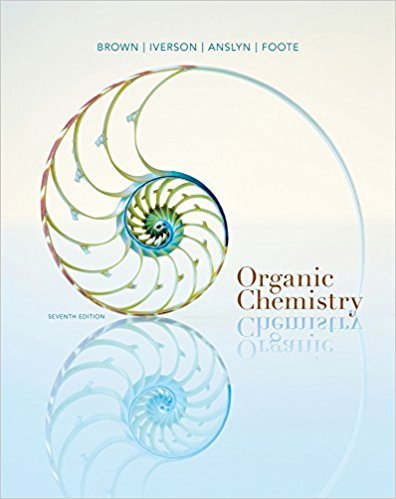×
Get Full Access to Organic Chemistry - 7 Edition - Chapter 20 - Problem 20.46
Get Full Access to Organic Chemistry - 7 Edition - Chapter 20 - Problem 20.46

×

# Show the product of the following reaction. Include stereochemistryISBN: 9781133952848 483

## Solution for problem 20.46 Chapter 20

Organic Chemistry | 7th Edition

• Textbook Solutions
• 2901 Step-by-step solutions solved by professors and subject experts
• Get 24/7 help from StudySoup virtual teaching assistantsOrganic Chemistry | 7th Edition

4 5 1 356 Reviews
21
5
Problem 20.46

Show the product of the following reaction. Include stereochemistry.

Step-by-Step Solution:
Step 1 of 3

Week Eight 8.1) ● Carbon dioxide amounts are growing ● Greenhouse gas effects → cause global warming 8.2) ● Chemical change → atoms rearrange and transform original substances into different. Ex. burning gasoline. Properties → corrosiveness, flammability, ductility, toxicity. ● Physical → Do not change composition. Ex. boiling water. Properties → Odor, taste, appearance, melting, boiling point, density. ● Chemical changes are evidenced by temperature and color change. 8.3) ● Chemical reactions are made of reactants on the left and products on the right. ● The equations must be balancesd ● Hint** Balance the most complex substance FIRST. 8.4) ● Coefficients in a chemical equation specify the relative amounts of moles of each of the substances involved in the reaction. ● Stoichiometry→ Relationship between chemical amounts. USE RATIOS ● Mass→ moles→ moles (ratio) → mass 8.5) ● Limiting reactant, Theoretical yield, Percent Yield. ● Limiting reactant → (reagent) completely consumed and limits the amount of product ● Reactant in excess → greater quantity than is required ● Theoretical Yield → product that can be made based on limiting reactant . ● Actual Yield → Actual amount of produced yielded. (actual/theoretical)*100% 8.6) ● Combustion reaction involves a substance and oxygen (diatomic molecule) and it emits heat, water, and carbon dioxide. ● Alkali → Reacts with halogens. More heat and stronger down group 1A ● Halogens → Make (metal halides), ionic bonds, also

Step 2 of 3

Step 3 of 3

## Discover and learn what students are asking

Calculus: Early Transcendental Functions : Exponential and Logarithmic Functions
?In Exercises 7-22, solve for $$x$$. $$3^{x}=81$$

Calculus: Early Transcendental Functions : Hyperbolic Functions
?In Exercises 7-14, verify the identity. $$\cosh ^{2} x=\frac{1+\cosh 2 x}{2}$$

Calculus: Early Transcendental Functions : Conservative Vector Fields and Independence of Path
?In Exercises 5 - 10, determine whether the vector field is conservative. $$\mathbf{F}(x, y)=e^{x}(\sin y \mathbf{i}+\cos y \mathbf{j})$$

Chemistry: The Central Science : Atoms, Molecules, and Ions
?Are these two compounds isomers? Explain. [Section 2.9]

Unlock Textbook Solution